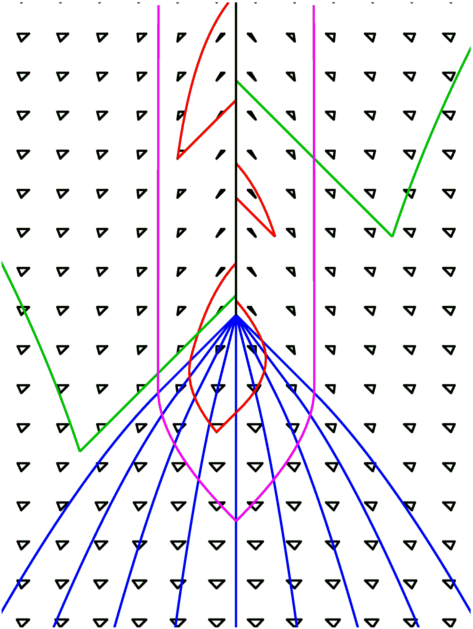# Formation of a Black HoleThe space-time diagram at right shows matter in blue collapsing to form a singularity [the solid black line], while the green curves are the future lightcones from events where light can escape to infinity, while the red curves are future lightcones from events where light cannot escape. The boundary between these two regions in space-time is the magenta curve. This boundary of the trapped region is the event horizon of the black hole. Note that the event horizon forms before the singularity and entirely surrounds it, so there is not a naked singularity in this space-time. The cosmic censorship hypothesis states that this is always true, but this hypothesis appears to be false in general, even though it is correct for this space-time and for all (or almost all) physically plausible space-times.

The little black triangles in the diagram are the local lightcones, and they trace out the metric structure of this space-time. The coordinates used in this diagram are the Kerr metric coordinates for times later than the creation of the singularity. The Kerr metric describes a rotating black hole, but the rotation is set to zero here. Before the creation of the singularity a simple analytic form is used instead of the exact solution for a collapsing sphere of pressureless matter.

Space-time is strongly curved near a black hole, so one has to be very careful about definitions. In particular, the radius of a black hole can not be defined as the distance from the central singularity to the event horizon, because observers trying to measure this would inevitably fall into the singularity. But if one measures the circumference of the event horizon and divides by 2*pi, one gets RS = 2GM/c2, which is called the Schwarzschild radius. Numerically it is 3 km for a 1 solar mass object. This is only a factor of a few smaller than the radius of a neutron star, which is 10 km for a 1.4 solar mass object.